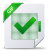# The Programming Language Oberon pdf

## The Programming Language Oberon pdf, tutoriel & guide de travaux pratiques en pdf.

Expressions

Expressions are constructs denoting rules of computation whereby constants and current values of variables are combined to derive other values by the application of operators and function procedures. Expressions consist of operands and operators. Parentheses may be used to express specific associations of operators and operands.

### Operands

With the exception of sets and literal constants, i.e. numbers and strings, operands are denoted by designators. A designator consists of an identifier referring to the constant, variable, or procedure to be designated. This identifier may possibly be qualified by module identifiers (see Ch. 4 and 11), and it may be followed by selectors, if the designated object is an element of a structure.
If A designates an array, then A[E] denotes that element of A whose index is the current value of the expression E. The type of E must be of type INTEGER. A designator of the form A[E1, E2, … , En] stands for A[E1][E2] … [En]. If p designates a pointer variable, p^ denotes the variable which is referenced by p. If r designates a record, then r.f denotes the field f of r. If p designates a pointer, p.f denotes the field f of the record p^, i.e. the dot implies dereferencing and p.f stands for p^.f.
The typeguard v(T0) asserts that v is of type T0, i.e. it aborts program execution, if it is not of type T0. The guard is applicable, if
1. T0 is an extension of the declared type T of v, and if
2.v is a variable parameter of record type, or v is a pointer.
designator = qualident {selector}.
selector = « . » ident | « [ » ExpList « ] » | « ^ » | « ( » qualident « ) ».
ExpList = expression {« , » expression}.
If the designated object is a variable, then the designator refers to the variable’s current value. If the object is a procedure, a designator without parameter list refers to that procedure. If it is followed by a (possibly empty) parameter list, the designator implies an activation of the procedure and stands for the value resulting from its execution. The (types of the) actual parameters must correspond to the formal parameters as specified in the procedure’s declaration (see Ch. 10).

#### Operators

The syntax of expressions distinguishes between four classes of operators with different precedences (binding strengths). The operator ~ has the highest precedence, followed by multiplication operators, addition operators, and relations. Operators of the same precedence associate from left to right. For example, x y z stands for (x y) z.
The available operators are listed in the following tables. In some instances, several different operations are designated by the same operator symbol. In these cases, the actual operation is identified by the type of the operands.
The operators +, , *, and / apply to operands of numeric types. Both operands must be of the same type, which is also the type of the result. When used as unary operators, denotes sign inversion and + denotes the identity operation.
The operators DIV and MOD apply to integer operands only. Let q = x DIV y, and r = x MOD y.
Relations are Boolean. The ordering relations <, <=, >, >= apply to the numeric types, CHAR, and character arrays. The relations = and # also apply to the types BOOLEAN and SET, and to pointer and procedure types. The relations <= and >= denote inclusion when applied to sets.

Introduction
2. Syntax
3. Vocabulary
4. Declarations and scope rules
5. Constant declarations
6. Type declarations
7. Variable declarations
8. Expressions
9. Statements
10. Procedure declarations
11. Modules
Appendix: The Syntax of OberonTélécharger le cours complet